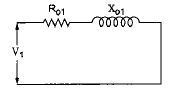Courses

# Test: D.C Machines & Transformers - 2

## 25 Questions MCQ Test Electrical Engineering SSC JE (Technical) | Test: D.C Machines & Transformers - 2

Description
This mock test of Test: D.C Machines & Transformers - 2 for Electrical Engineering (EE) helps you for every Electrical Engineering (EE) entrance exam. This contains 25 Multiple Choice Questions for Electrical Engineering (EE) Test: D.C Machines & Transformers - 2 (mcq) to study with solutions a complete question bank. The solved questions answers in this Test: D.C Machines & Transformers - 2 quiz give you a good mix of easy questions and tough questions. Electrical Engineering (EE) students definitely take this Test: D.C Machines & Transformers - 2 exercise for a better result in the exam. You can find other Test: D.C Machines & Transformers - 2 extra questions, long questions & short questions for Electrical Engineering (EE) on EduRev as well by searching above.
QUESTION: 1

Solution:
QUESTION: 2

Solution:
QUESTION: 3

### When voltage is transformed from primary to secondary then it is _____.

Solution:
QUESTION: 4

If the applied voltage to a transformer primary isincreased by keeping the V/f ratio fixed, then the magnetizing current and the core loss will,respectively:

Solution:
QUESTION: 5

The applied voltage of a certain transformer isincreased by 75%; while the frequency of the applied voltage is reduced by 25%. The maximum core flux density will :

Solution:
QUESTION: 6

On no-load phasor diagram of transformer, thecore loss component of the current remains in phase with

Solution:
QUESTION: 7

At which condition of the transformer theequivalent circuit will be as shown in the figure ?Solution:
QUESTION: 8

In a transformer, zero voltage regulation at full load s:

Solution:
QUESTION: 9

While performing the open-circuit and shortcircuit tests on a transformer to determine parameters the status of the low voltage (LV) and high voltage (HV) windings will be such that

Solution:
QUESTION: 10

A 2 kVA transformer has iron loss of 150 Wand full-load copper loss of 250 W. Themaximum efficiency of the transformer wouldoccur when the total loss is

Solution:
QUESTION: 11

Grain oriented laminated sheet steel intransformer reduces

Solution:
QUESTION: 12

The colour of fresh dielectric oil used intransformers is

Solution:
QUESTION: 13

Transformer oil must be free from

Solution:
QUESTION: 14

Conservator (for transformer) is used

Solution:
QUESTION: 15

The noise, in a transformer, caused by vibrationsof laminations set by magnetic forces, is termed as

Solution:
QUESTION: 16

Distribution transformers have core losses

Solution:
QUESTION: 17

In Scott connection, if the turns ratio of main transformer is K,

Solution:
QUESTION: 18

The copper loss is constant for

Solution:
QUESTION: 19

Current-versus time graph for an idealcommutation is a

Solution:
QUESTION: 20

The dummy coils in d.c. machines re useful to

Solution:
QUESTION: 21

In a series wound d.c. machine (generator ormotor), the series field winding consists of

Solution:
QUESTION: 22

A d.c. shunt motor takes 5A at 100 V whenrunning light. shunt field resistance is 50W and armature resistance is 0.2W. What is the driving power ?

Solution:
QUESTION: 23

If the applied voltage to a dc machine is 230 V, then the back emf, for maximum power developed, is

Solution:
QUESTION: 24

The armature resistance of a 6-pole lap woundgenerator is 0.05W. If the armature is rewoundusing a wave winding, the armature resistancewill be

Solution:
QUESTION: 25

A 4-pole, 500 V shunt motor has 720 wave connected conductors on its armature. The fullloadarmature current is 60 A and flux per poleis 0.03 Wb. The armature resistance is 0.2Wand contact drop per brush is 1V. What is the back e.m.f ?

Solution: